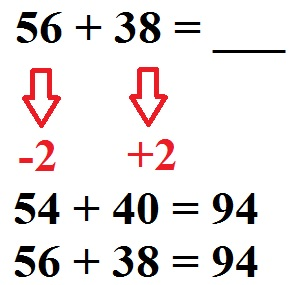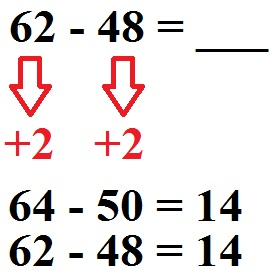# What is meant by subtraction and addition

• A Explanationwhat beneficial arithmetic is all about.
• Lots Examples to use computational advantages.
• tasks / Exercises so that you can practice this topic yourself.
• Some Videos to calculate with numbers.
• A Question and answer area around this topic.

In order to be able to use computational advantages at all, you should already be able to do simple additions and subtractions (i.e. plus and minus), multiplications (take times) and divisions (dividing). If you still have problems here, please read these topics first and deal with the related tasks.

The idea of ​​computational advantages is actually quite simple:

Note:
Mathematical advantage is about solving a task in the simplest possible way. To do this, you have to resort to "tricks" from time to time in order to be able to solve the task easily. In some cases, laws of calculation have to be applied.

Sometimes you have problems calculating a task with larger numbers. One possibility is then to simplify the task and first solve the simplified task. For 84 + 2 this should be shown once with an addition:

• Big task: 84 + 2 = 86
• Small task: 4 + 2 = 6

Here you leave out the tens digit for now. We first turn 84 + 2 into 4 + 2. This results in 6. Then we add the 8 from the 84. With this we can solve the big problem 84 + 2 = 86.

The idea with the small task can also be used for subtraction. Here, too, we take out the tens for the small exercise, so only count with the ones. Let's look at this for 18-4:

• Big task: 18 - 4 = 14
• Small task: 8 - 4 = 4

Another way to use arithmetic advantages is to change a task so that you can calculate with the numbers more easily. Let's do this once with 56 + 38.At 56 + 38, some may have problems. But what changes in the result if I turn it into 54 + 40? Nothing. So we do this by subtracting 2 once and adding 2 once. The calculation of 54 + 40 = 94 should be easier for many people.

Of course, this also works with subtraction. To see at 62 - 48:Here we add 2 each to get 64 - 50. The result 14 then applies to the simplified task as well as to the original task.

3. Commutative law, associative law

Next, let's look at a law of calculation. The commutative law can be used for additions and also for multiplications. In German this is often referred to as the law of exchange. This also makes sense, because you can swap the numbers during addition and multiplication without changing anything in the result. Some find it easier to solve a problem after swapping them. With the associative law, the sum-mands can be combined as desired.

For the multiplication:

This sometimes results in computational advantages:

• 12 + 18 + 8 + 2 = ____
• 12 + 8 + 18 + 2 = ____
• 20 + 20 = 40

In this case you can rearrange the numbers in such a way that each package contains 20 and it is easier to get the 40 as the total.

Note:

Important: The commutative law does not apply to subtraction (calculate minus) and division (divide). So you can't use it here!

4. Distributive law

With the distributive law, a constant factor can be excluded.

• a * b + a * c = a · (b + c)

Means for example:

• a = 3
• b = 8
• c = 5
• 3 · 8 + 3 · 5 = 39
• 3 · (8 + 5) = 39

• 3 · 5 + 3 · 6 + 3 · 7 = ____
• 3 · (5 + 6 + 7) = ____
• 3 · 18 = 54
Display:

In this section a few more examples of advantageous calculations are to be shown.

example 1:

Use arithmetic advantages to calculate the problem 88 + 112.

Solution:

• 88 + 112 = ____
• 90 + 110 = 200

Example 2:

Solve 73 + 98 + 27 + 2 in the simplest possible way.

• 73 + 98 + 27 + 2 = ____
• 73 + 27 + 98 + 2 = 200

Example 3:

Use arithmetic advantages to solve 7 8 + 3 8 + 5 8.

Solution:

• 7 · 8 + 3 · 8 + 5 · 8 = ____
• 8 · (7 + 3 + 5) = ____
• 8 · 15 = 120

Now it's your turn. Turns our tasks or exercises into arithmetic advantages (advantageous arithmetic) and tries to come up with the right results yourself.

Show:

### Examples and explanations

The next video is about advantageous arithmetic. For this purpose, I will give you various examples and tasks for the basic arithmetic operations of addition, subtraction, multiplication and division.

Next video »